# Wasserstein metric

(diff) ← Older revision | Latest revision (diff) | Newer revision → (diff)

Vasershtein metric

The "Wasserstein metric" has a colourful history with several quite different fields of applications. It also has various historical sources.

The term "Vasershtein distance" appeared for the first time in [a3]. For probability measures,(cf. also Probability measure) on a metric space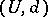, L.N. Vasershtein [a10] had introduced the metric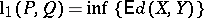, where the infimum is with respect to all random variables,with distributions,. His work was very influential in ergodic theory in connection with generalizations of the Ornstein isomorphism theorem (see [a5]). In the English literature the Russian name was pronounced typically as "Wasserstein" and the notationis common for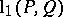.

The minimal-metrichad been introduced and investigated already in 1940 by L.V. Kantorovich for compact metric spaces [a6]. This work was motivated by the classical Monge transportation problem. Subsequently, the transportation distance was generalized to general cost functionals; the special case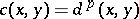leads to the minimal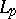-metric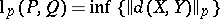, with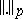the usual-norm. The famous Kantorovich–Rubinshtein theorem [a8] gives a dual representation of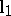in terms of a Lipschitz metric: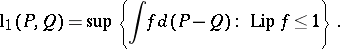From this point of view, the notion of a Kantorovich metric or minimal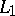-metric (or minimal-metric) seems historically to be also appropriate.

In fact, in 1914, C. Gini, while introducing a "simple index of dissimilarity" , also defined themetric in a discrete setting on the real line and T. Salvemini (the discrete case, [a9]) and G. Dall'Aglio (the general case, [a2]) proved the basic representation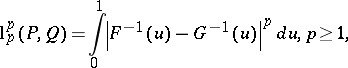where,are the distribution functions of,. Gini had given this formula for empirical distributions and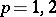. This influential work initiated a lot of work on measures with given marginals in the Italian School of probability, while M. Fréchet [a4] explicitly dealt with metric properties of these distances.

C.L. Mallows [a7] introduced the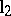-metric in a statistical context. He used its properties for proving a central limit theorem and proved the representation above. Based on Mallows work, P.J. Bickel and D.A. Freedman [a1] described topological properties and investigated applications to statistical problems such as the bootstrap (cf. also Bootstrap method). They introduced the notion of a Mallows metric for. This notion is used mainly in the statistics literature and in some literature on algorithms.

So, the minimal-metricwas invented historically several times from different perspectives. Maybe historically the name Gini–Dall'Aglio–Kantorovich–Vasershtein–Mallows metric would be correct for this class of metrics. For simplicity reasons it seems preferable to use the notion of a minimal-metric, and write it as.

How to Cite This Entry:
Wasserstein metric. Encyclopedia of Mathematics. URL: http://encyclopediaofmath.org/index.php?title=Wasserstein_metric&oldid=15624
This article was adapted from an original article by L. Rueshendorff (originator), which appeared in Encyclopedia of Mathematics - ISBN 1402006098. See original article